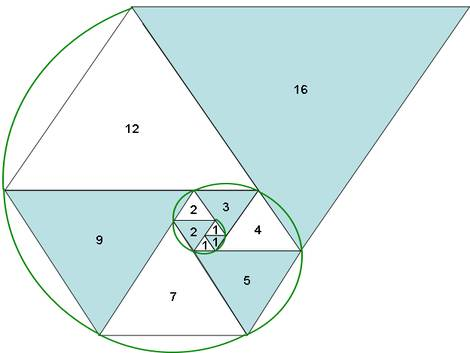# The Padovan sequence

#### PHP Script that demons,trate the Padovan sequence

Date: 6-jun-2010

The Padovan Saquence is a set of integers that has interesting properties. To construct this sequece we are going to initialize the three first item in our vector to 1.
So,

P=1;
P=1;
P=1;

And then apply the equation:
P[i]=P[i-2] + P[i-3]

Here is the code:

`/** * Simple script to demonstrate the Padovan sequence * having the equation P[i]=P[i-2] + P[i-3] * where P=P=P=1 */ /* --- Initialize the three first values to 1 in the vector */ \$P=1; \$P=1; \$P=1;  \$i=3; \$numResults=21; do {    /* --- Apply equation P[i]=P[i-2] + P[i-3] */    \$P[\$i]=\$P[\$i-2] + \$P[\$i-3];    \$i++; } while (\$i<\$numResults);   /* --- Print values */ for(\$i=0;\$i<=\$numResults;\$i++) {    echo "\n".\$P[\$i]; }  /*Will print:11122345791216212837496586114151200*/`

Now look at this image, it is a spiral that follow the Padovan sequence:This image proofs the property:

P(n) = P(n − 1) + P(n − 5)

You can see it clearly the triangle sized 9 is equel to the addition of the two side triangles (7 + 2)

You can check all this in Wiki